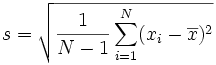# Standard Deviation Calculator

Here are the step-by-step calculations to work out the Standard Deviation (see below for formulas).

When your data is the whole population the formula is:(The "Population Standard Deviation")

When your data is a sample the formula is:(The "Sample Standard Deviation")

The important difference is "N-1" instead of "N" ... read more at Standard Deviation Formulas.